SLVSBY7 February   2017

PRODUCTION DATA.

1. Features
2. Applications
3. Description
4. Revision History
5. Device Comparison Table
6. Pin Configuration and Functions
7. Specifications
8. Detailed Description
1. 8.1 Overview
2. 8.2 Functional Block Diagram
3. 8.3 Feature Description
4. 8.4 Device Functional Modes
5. 8.5 Register Maps
9. Application and Implementation
10. 10Power Supply Recommendations
11. 11Layout
12. 12Device and Documentation Support
13. 13Mechanical, Packaging, and Orderable Information

• RWF|72

## 7 Specifications

### 7.1 Absolute Maximum Ratings

over operating free-air temperature range (unless otherwise noted)(1)
MIN MAX UNIT
Input voltage PVDD_x/AVDD to PBKG -0.3 40 V
PVSS_x/REFGND/DCDC_AGND_x/DAC_AGND_x to PBKG -0.3 0.3
VPOS_IN_x to VNEG_IN_x -0.3 40
VPOS_IN_x to PBKG -0.3 33
VNEG_IN_x to PBKG -20 0.3
VSENSEN_x to PBKG VNEG_IN_x VPOS_IN_x
VSENSEP_x to PBKG VNEG_IN_x VPOS_IN_x
DVDD to PBKG -0.3 6
REFOUT/REFIN to PBKG -0.3 6
Digital input voltage to PBKG -0.3 DVDD+0.3
Output voltage VOUT_x to PBKG VNEG_IN_x VPOS_IN_x V
IOUT_x to PBKG VNEG_IN_x VPOS_IN_x
SDO, ALARM to PBKG -0.3 DVDD+0.3
Input current Current into any digital input pin -10 10 mA
Power dissipation (TJmax – TA)/θJA W
Operating junction temperature, TJ -40 150 °C
Junction temperature range, TJmax 150
Storage temperature, Tstg -65 150
Stresses beyond those listed under Absolute Maximum Ratings may cause permanent damage to the device. These are stress ratings only, which do not imply functional operation of the device at these or any other conditions beyond those indicated under Recommended Operating Conditions. Exposure to absolute-maximum-rated conditions for extended periods may affect device reliability.

### 7.2 ESD Ratings

VALUE UNIT
V(ESD) Electrostatic discharge Human body model (HBM), per ANSI/ESDA/JEDEC JS-001, all pins(1) ±2000 V
Charged device model (CDM), per JEDEC specification JESD22-C101, all pins(2) ±500
JEDEC document JEP155 states that 500-V HBM allows safe manufacturing with a standard ESD control process.
JEDEC document JEP157 states that 250-V CDM allows safe manufacturing with a standard ESD control process.

### 7.3 Recommended Operating Conditions

over operating free-air temperature range (unless otherwise noted)
MIN NOM MAX UNIT
POWER SUPPLY
PVDD_x/AVDD_x to PBKG/PVSS_x(1) Positive supply voltage to ground range 12 36 V
VPOS_IN_x to PBKG(1) Positive supply voltage to ground range 12 33 V
VNEG_IN_x to PBKG(1) Negative supply voltage to substrate for current output mode -18 0 V
Negative supply voltage to substrate for voltage output mode -18 -5 V
VPOS_IN_x to VNEG_IN_x(1) 12 36 V
VSENSEN_x to PBKG The minimum headroom spec for voltage output stage must be met -7 7 V
DVDD to PBKG Digital supply voltage to substrate 2.7 5.5 V
DIGITAL INPUTS
VIH Input high voltage 2 V
VIL Input low voltage 0.6 V
REFERENCE INPUT
REFIN to PBKG Reference input to substrate 4.95 5.05 V
TEMPERATURE RANGE
TA Operating temperature -40 125 °C
The minimum headroom spec for voltage output stage and the compliance voltage for current output stage should be met. When Buck-Boost converter is enabled VPOS_IN_x/VNEG_IN_x are generated internally to meet headroom and compliance specs. When Buck-Boost converter is disabled VPOS_IN_x, AVDD, and PVDD must be tied together.

### 7.4 Thermal Information

THERMAL METRIC(1) DAC8775 UNIT
RWF (VQFN)
72 PINS
RθJA Junction-to-ambient thermal resistance 21.7 °C/W
RθJC(top) Junction-to-case (top) thermal resistance 3.3 °C/W
RθJB Junction-to-board thermal resistance 1.9 °C/W
ΨJT Junction-to-top characterization parameter 0.1 °C/W
ΨJB Junction-to-board characterization parameter 1.9 °C/W
RθJC(bot) Junction-to-case (bottom) thermal resistance 0.2 °C/W

### 7.5 Electrical Characteristics

AVDD/PVDD_x/VPOS_IN_x = +15 V, VNEG_IN_x = -15 V, VSENSEN_x = PBKG = PVSS_x = 0 V, External DVDD = 2.7 V. VOUT : RL = 1 kΩ, CL = 200 pF, IOUT : RL = 250 Ω; all specifications -40℃ to +125℃, unless otherwise noted. REFIN= +5 V external;, Buck-Boost Converter disabled unless otherwise stated
PARAMETER TEST CONDITIONS MIN TYP MAX UNIT
CURRENT OUTPUT
IOUT Output Current Ranges 0 24 mA
0 20 mA
3.5 23.5 mA
-24 24 mA
4 20 mA
Accuracy
Resolution 16 Bits
INL Relative Accuracy(1) All ranges except bipolar range -12 12 LSB
Bipolar range only -16 16 LSB
DNL Differential Nonlinearity(1) Ensured monotonic -1 1 LSB
TUE Total Unadjusted Error(1) -40℃ to +125℃ -0.14 0.14 %FSR
-40℃ to +125℃, 4 to 20 mA -0.4 0.4 %FSR
TA = +25℃, 4 to 20 mA -0.2 0.2 %FSR
TA = +25°C -0.12 0.12 %FSR
OE Offset Error(1) -40℃ to +125℃ -0.1 0.1 %FSR
TA = +25°C -0.05 0.05 %FSR
OE-TC Offset Error Temperature Coefficient -40℃ to +125℃ 4 ppm FSR/ºC
ZCE Zero Code Error -40℃ to +125℃, 0x0000h into DAC -15 15 uA
-40℃ to +125℃, 0x0000h into DAC, 4 to 20 mA -18 18 uA
TA = 25℃, 0x0000h into DAC 1.2 m%FSR
TA = 25℃, 0x0000h into DAC, 4 to 20 mA 1.8 m%FSR
ZCE-TC Zero Code Error Temperature Coefficient 0x0000h into DAC, -40℃ to +125℃ 4 ppm/ºC
GE Gain Error(2) -40℃ to +125℃ -0.125 0.125 %FSR
-40℃ to +125℃, 4 to 20 mA -0.25 0.25 %FSR
TA = +25℃, 4 to 20 mA -0.2 0.2 %FSR
TA = +25°C -0.12 0.12 %FSR
GE-TC Gain Error Temperature Coefficient -40℃ to +125℃ 3 ppm FSR/ºC
PFSE Positive Full Scale Error 0xFFFFh into DAC, -40℃ to +125℃ -0.125 0.125 %FSR
0xFFFFh into DAC, -40℃ to +125℃, 4 to 20 mA -0.25 0.25 %FSR
0xFFFFh into DAC, TA = 25℃ 0.016 %FSR
0xFFFFh into DAC, TA = 25℃, 4 to 20 mA 0.024 %FSR
NFSE Negative Full Scale Error 0x0000h into DAC, Bipolar range only, -40℃ to +125℃ -0.125 0.125 %FSR
0x0000h into DAC, Bipolar range only, TA = 25℃ 0.02 %FSR
PFSE-TC Positive Full Scale Error Temperature Coefficient 5 ppm FSR/ºC
NFSE-TC Negative Full Scale Error Temperature Coefficient Bipolar range only 5 ppm FSR/ºC
BPZE Bipolar Zero Error Bipolar range only, 0x8000h into DAC -40℃ to +125℃ -0.05 0.05 %FSR
Bipolar range only, 0x8000h into DAC, TA = +25°C -0.02 0.02
BPZE-TC Bipolar Zero Error Temperature Coefficient 0x8000h into DAC,-40℃ to +125℃ 4 ppm/ºC
VCL Compliance Voltage Output = 24 mA VPOS_IN_x-3 V
Output = ±24 mA |VNEG_IN_x|+3 VPOS_IN_x-3 V
RL Resistive Load All except ±24 mA range 1.2
±24 mA range 0.625
DC-PSRR DC Power Supply Rejection Ratio Code = 0x8000, 20 mA range 0.1 µA/V
ZO Output Impedance Code = 0x8000 10
IOLEAK Output Current Leakage Iout is disabled or in power-down 1 nA
HART INTERFACE
VHART-IN HART Input 400 500 600 mVpp
Corresponding Output HART In = 500 mVpp 1.2 KHz 1 mApp
VOLTAGE OUTPUT
VOUT Voltage Output Ranges (normal mode) 0 5 V
0 10 V
-5 5 V
-10 10 V
Voltage Output Ranges (Overrange mode) 0 6 V
0 12 V
-6 6 V
-12 12 V
Accuracy
Resolution 16 Bits
INL Relative Accuracy, INL(1) -12 12 LSB
DNL Differential Nonlinearity, DNL(1) Ensured monotonic -1 1 LSB
TUE Total Unadjusted Error, TUE(1) -40℃ to +125℃, VOUT unloaded -0.1 ±0.05 0.1 %FSR
TA = +25°C, VOUT unloaded -0.075 0.075 %FSR
ZCE Zero Code Error(5) Unipolar ranges only, VOUT unloaded, -40℃ to +125℃ -2.5 2.5 mV
Unipolar ranges only, VOUT unloaded, TA = 25℃ 0.14 mV
ZCE-TC Zero Code Error Temperature Coefficient Unipolar ranges only, -40℃ to +125℃ 2 ppm FSR/ºC
BPZE Bipolar Zero Error Bipolar range only, 0x8000h into DAC -40℃ to +125℃, VOUT unloaded -0.03 0.03 %FSR
Bipolar range only, 0x8000h into DAC, TA = +25°C, VOUT unloaded -0.025 0.025 %FSR
BPZE-TC Bipolar Zero Error Temperature Coefficient Bipolar range only, 0x8000h into DAC, -40℃ to +125℃, VOUT unloaded 1 ppm FSR/ºC
GE Gain Error(1) -40℃ to +125℃, VOUT unloaded -0.1 0.1 %FSR
TA = +25°C, VOUT unloaded -0.07 0.07 %FSR
GE-TC Gain Error Temperature Coefficient -40℃ to +125℃ 3 ppm FSR/ºC
PFSE Positive Full Scale Error 0xFFFFh into DAC, -40℃ to +125℃, VOUT unloaded -0.1 0.1 %FSR
0xFFFFh into DAC, TA = 25℃, VOUT unloaded 0.03 %FSR
NFSE Negative Full Scale Error(5) Bipolar ranges only, 0x0000h into DAC, -40℃ to +125℃, VOUT unloaded -0.06 0.06 %FSR
Bipolar ranges only, 0x0000h into DAC, TA = 25℃, VOUT unloaded 0.002 %FSR
PFSE-TC Positive Full Scale Error Temperature Coefficient VOUT unloaded, -40℃ to +125℃ 2 ppm FSR/ºC
NFSE-TC Negative Full Scale Error Temperature Coefficient VOUT unloaded, -40℃ to +125℃ 2 ppm FSR/ºC
Output unloaded, VPOS_IN_x with respect to VOUT_x, 0xFFFFh into DAC, 1 kΩ load 3 V
Footroom Bipolar, ranges only, VNEG_IN_x with respect to VOUT_x, 0x0000h into DAC 3 V
Unipolar ranges only, VNEG_IN_x with respect to VOUT_x, 0x0000h into DAC 5 V
Short-Circuit Current SCLIM[1:0] = "00" (see register map) 17 23 mA
SCLIM[1:0] = "01" (see register map) 8 11 mA
SCLIM[1:0] = "10" (see register map) 22 28 mA
SCLIM[1:0] = "11" (see register map) 26 34 mA
CL Capacitive Load Stability RL = Open 20 nF
RL = 1 kΩ 20 nF
RL = 1 kΩ with External compensation capacitor (150 pF) connected 1 µF
ZO DC Output Impedance Voltage output enabled, VOUT = Mid Scale, UP10V range 0.01 Ω
Voltage output disabled (POC = '1') 50
Voltage output disabled (POC = '0') 30
ILEAK Output Leakage (VOUT_x Pin) Voltage output disabled (POC = '1') 1 nA
DC-PSRR DC Power Supply Rejection Ratio No output load 10 µV/V
VSENSEP Impedance VOUT enabled Mid-Scale UP10 240
VSENSEN Impedance VOUT enabled Mid-Scale UP10 120
EXTERNAL REFERENCE INPUT
IREF External Reference Current VOUT = Full scale, BP12V range, per channel 0.35 mA
Reference Input Capacitance 100 pF
INTERNAL REFERENCE OUTPUT
VREF Reference Output TA = 25°C 4.99 5.01 V
VREF-TC Reference TC TA = -40℃ to +125℃ -13 13 ppm/°C
TA = -25℃ to +125℃ -10 10 ppm/°C
TUE DAC Voltage Output Total Unadjusted Error(1) -40°C to +125°C, VOUT_x unloaded, Internal reference enabled 0.2 %FSR
DAC Current Output Total Unadjusted Error(1) -40°C to +125°C, Internal reference enabled 0.2 %FSR
-40°C to +125°C, Internal reference enabled, 4 mA to 20 mA range 0.5 %FSR
Output Noise (0.1 Hz to 10 Hz) TA = 25°C 13 µV p-p
Noise Spectral Density At 10 kHz, At 25°C 200 nV/sqrtHz
Short Circuit Current Ref-Out shorted to PBKG 20 mA
Load Regulation Sourcing and Sinking, TA = +25°C 5 µV/mA
Line Regulation TA = +25°C 2 uV/V
BUCK BOOST CONVERTER
RON Switch On Resistanvce TA = +25°C 3 Ω
ILEAK Switch Leakage Current TA = +25°C 20 nA
L Inductor Between LP_x and LN_x 100 µH
ILMAX Peak Inductor Current TA = +25°C, PVDD = AVDD = 36 V, Buck-Boost Converter enabled 0.5 A
VO Output Voltage VPOS_IN_x minimum 4 V
VPOS_IN_x maximum 32 V
VO Output Voltage VNEG_IN_x minimum -18 V
VNEG_IN_x maximum -5 V
CL Load Capacitor VPOS_IN_x and VNEG_IN_x 10 µF
Start Up Time After enabling VPOS_IN_x and VNEG_IN_x with 10 µF load capacitor on these pins 3 ms
DVDD LDO
VO Output Voltage 5 V
THERMAL ALARM
Trip Point 150 °C
Hysteresis 15 °C
DIGITAL INPUTS
Hysteresis Voltage 0.4 V
Input Current -5 5 µA
Input Current (DVDD_EN) -10 10 µA
Pin Capacitance Per pin 10 pF
DIGITAL OUTPUTS
SDO
VOL Output Low Voltage Sinking 200 µA 0.4 V
VOH Output High Voltage Sourcing 200 µA DVDD-0.5 V
ILEAK High Impedance Leakage -5 5 µA
High Impedance Output Capacitance 10 pF
ALARM
VOL Output Low Voltage At 2.5 mA 0.4 V
ILEAK High Impedance Leakage 50 µA
High Impedance Output Capacitance 10 pF
POWER REQUIREMENTS
IAVDD+IPVDD Current Flowing into AVDD and PVDD All Buck-Boost converter positive output enabled, IOUT_x mode operation, All IOUT channels enabled, 0 mA, PVDD = AVDD = 12 V, Internal reference, VNEG_IN_x = 0 V 5 mA
All IOUT Active, 0 mA, 0 to 24 mA range, VNEG_IN_x = 0 V 3.5 5 mA
IPVDD_x Current Flowing into PVDD Buck-Boost converter enabled, Peak current 0.5 A
Buck-Boost converter disabled 0.1 mA
IDVDD Current Flowing into DVDD All digital pins at DVDD, DVDD = 5.5 V 1.8 mA
IVPOS_IN_x Current Flowing into VPOS_IN_x IOUT active, 0 mA, 0 to 24 mA range 1.2 mA
VOUT active, No load, 0  to 10 V range, Mid scale code 3 mA
IVNEG_IN_x Current Flowing into VNEG_IN_x IOUT active, 0 mA, ±24 mA range 1.2 mA
VOUT active, No load, 0 to 10 V range, Mid scale code 3 mA
PDISS Power Dissipation (PVDD+AVDD) All Buck-Boost converter positive output enabled, IOUT_x mode operation, All IOUT channels enabled, Rload = 1 Ω, 24 mA, PVDD = AVDD = 12 V, Internal reference, VNEG_IN_x = 0 V 0.86 1.1 W
IVSENSEP Current Flowing into VSENSEP VOUT disabled 40 nA
IVSENSEN Current Flowing into VSENSEN VOUT disabled 20 nA
DYNAMIC PERFORMANCE
Voltage Output
Tsett Output Voltage Settling Time 0 to10 V, to ±0.03% FSR RL = 1K||CL = 200 pF 15 µs
0 to 5 V, to ±0.03% FSR RL = 1K||CL = 200 pF 10 µs
-5 to 5 V, to ±0.03% FSR RL = 1K||CL = 200 pF 15 µs
-10 to 10 V, to ±0.03% FSR RL = 1K||CL = 200 pF 30 µs
Output Voltage Ripple Buck-Boost converter enabled, 50 KHz, 20dB/decade filter on VPOS_IN_x 2 mVpp
SR Slew Rate RL = 1K||CL = 200 pF 1 V/µs
Power-On Glitch Magnitude(2) 0.1 V
Power-off Glitch Magnitude(3) 0.8 V
Channel to Channel DC Crosstalk Full scale swing on adjacent channel 2 m%FSR
Code-to-Code Glitch 0.15 µV-sec
Digital Feedthrough 1 nV-sec
Output Noise (0.1 Hz to 10 Hz bandwidth) UP10V, Mid scale 0.1 LSB p-p
Output Noise (100 kHz bandwidth) UP10V, Mid scale 200 µVrms
Output Noise Spectral Density BP20V Measured at 10 kHz, Mid scale 200 nV/sqrtHz
AC-PSRR AC Power Supply Rejection Ratio 200 mV 50/60Hz Sine wave superimposed on power supply voltage. (AC analysis) -75 dB
Current Output
Tsett Output Current Settling Time 24 mA Step, to 0.1% FSR, no L 10 µs
24 mA Step, to 0.1% FSR , L = 1 mH, CL = 22 nF 50 µs
Output Current Ripple Buck-Boost converter enabled, 50 KHz, 20dB/decade filter on VPOS_IN_x 8 µApp
AC-PSRR AC Power Supply Rejection Ratio 200 mV 50/60Hz Sine wave superimposed on power supply voltage. -75 dB
For current output all ranges except ±24 mA, low code of 256d and a high code of 65535d are used, for ±24 mA range low code of 0d and a high code of 65535d. For voltage output, low code of 256d and a high code of 65535d are used
No load, DVDD supply ramps up before VPOS_IN_x,and VNEG_IN_x, ramp rate of VPOS_IN_x,and VNEG_IN_x limited to 18 V/msec
Vout disabled, no load, ramp rate of VPOS_IN_x,and VNEG_IN_x limited to 18 V/msec
680 nF is required at IOUT pin for 50 mH pure inductor load.
DAC code at 0d, this error includes offset error of the DAC since the DAC is linear between 0d to 65535d

### 7.6 Timing Requirements: Write and Readback Mode

At TA = –40°C to +125°C and DVDD = +2.7 V to +5.5 V, unless otherwise noted.
PARAMETER TEST CONDITIONS MIN MAX UNIT
fSCLK Max clock frequency 25 MHz
t1 SCLK cycle time 40 ns
t2 SCLK high time 18 ns
t3 SCLK low time 18 ns
t4 SYNC falling edge to SCLK falling edge setup time 15 ns
t5 24th/32nd SCLK falling edge to SYNC rising edge 13 ns
t6 SYNC high time 40 ns
t7 Data setup time 8 ns
t8 Data hold time 5 ns
t9 SYNC rising edge to LDAC falling edge 33 ns
t10 LDAC pulse width low 10 ns
t11 LDAC falling edge to DAC output response time 50 ns
t12 DAC output settling time See Electrical Characteristics µs
t13 CLR high time 10 ns
t14 CLR activation time 50 ns
t15 SCLK rising edge to SDO valid 14 ns
t16 SYNC rising edge to DAC output response time 50 ns
t17 LDAC falling edge to SYNC rising edge 100 ns
t18 RESET pulse width 10 ns
t19 SYNC rising edge to CLR falling/rising edge 60 ns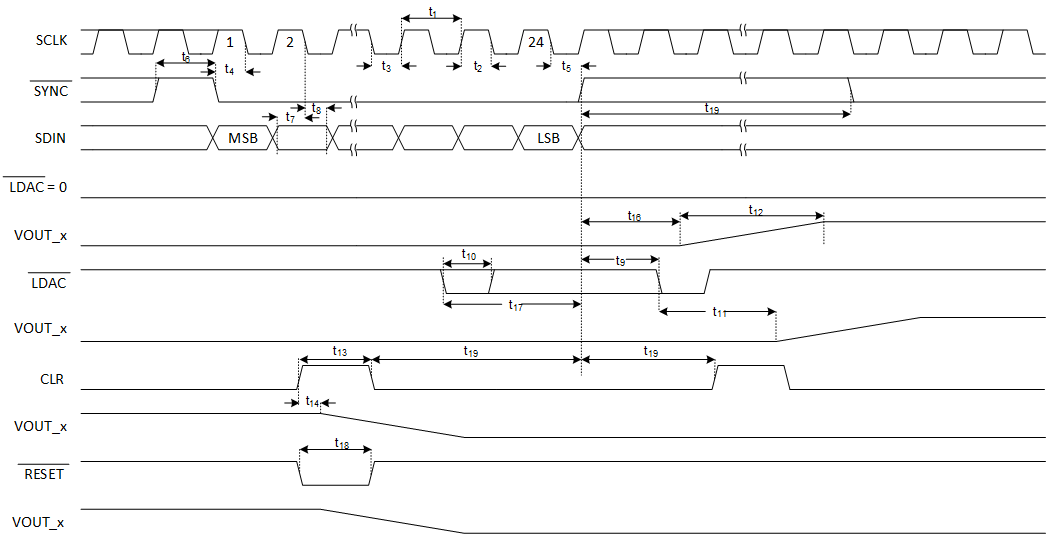Figure 1. Write Mode TimingFigure 2. Readback Mode Timing

### 7.7 Typical Characteristics

AVDD/PVDD_x/VPOS_IN_x = +15 V, VNEG_IN_x = –15 V, PBKG = PVSS_x = 0 V, External DVDD = 5 V, VOUT disabled, IOUT RL = 250 Ω, TA = 25℃, REFIN = +5 V external, Buck-Boost Converter disabled, unless otherwise stated.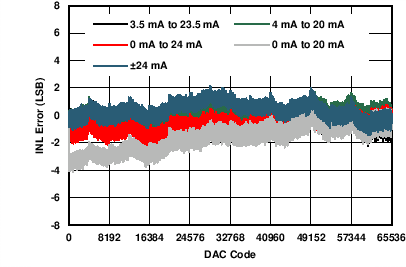Figure 3. IOUT Linearity Error vs Digital Input Code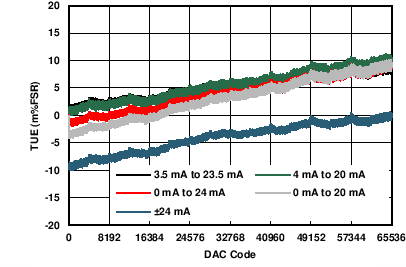Figure 5. IOUT Total Unadjusted Error vs Digital Input CodeFigure 7. IOUT Differential Linearity Error vs Temperature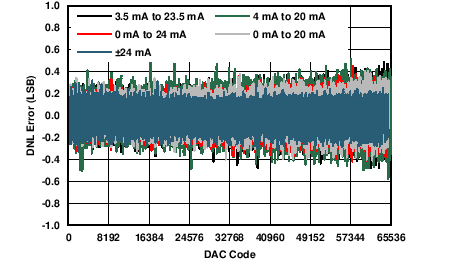Figure 4. IOUT Differential Linearity Error vs Digital Input CodeFigure 6. IOUT Linearity Error vs TemperatureFigure 8. IOUT Total Unadjusted Error vs Temperature
AVDD/PVDD_x/VPOS_IN_x = +15 V, VNEG_IN_x = –15 V, PBKG = PVSS_x = 0 V, External DVDD = 5 V VOUT disabled, IOUT RL = 250 Ω, TA = 25℃, REFIN= +5 V external, Buck-Boost Converter disabled, unless otherwise stated.Figure 9. IOUT Offset Error vs Temperature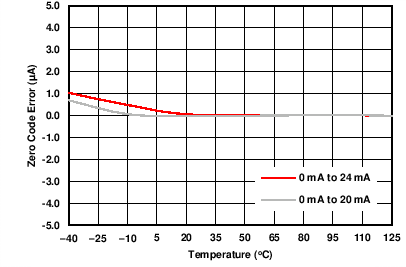Figure 11. IOUT Zero Code Error vs TemperatureFigure 13. IOUT Bipolar Zero Error vs TemperatureFigure 10. IOUT Gain Error vs Temperature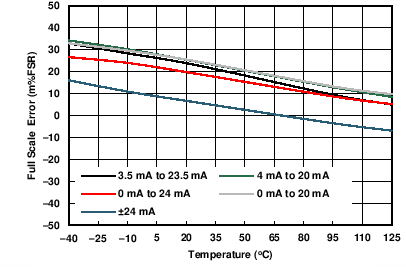Figure 12. IOUT Full Scale Error vs Temperature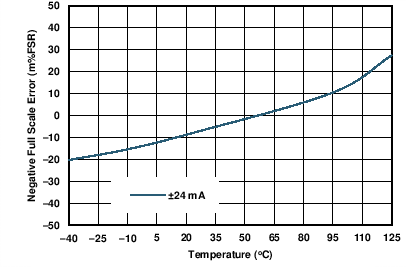Figure 14. IOUT Negative Full Scale Error vs Temperature
AVDD/PVDD_x = VPOS_IN_x , VNEG_IN_x = PBKG = PVSS_x = 0 V, External DVDD = 5 V VOUT disabled, IOUT RL = 250 Ω, TA = 25℃, REFIN = +5 V external, Buck-Boost Converter disabled, unless otherwise stated.Figure 15. IOUT Linearity Error vs Power Supplies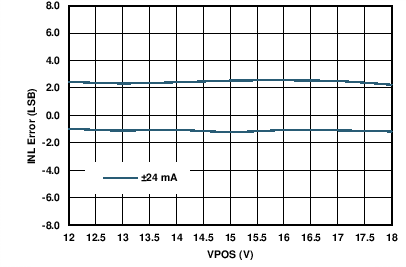|VPOS_IN_x| = |VNEG_IN_x|
Figure 17. IOUT Linearity Error vs Power Supplies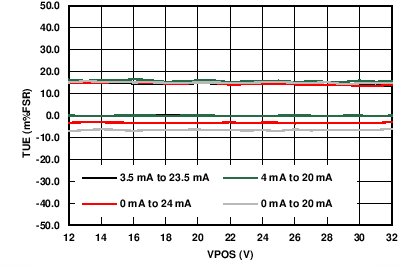Figure 19. IOUT Total Unadjusted Error vs Power SuppliesFigure 16. IOUT Differential Linearity Error vs Power Supplies|VPOS_IN_x| = |VNEG_IN_x|
Figure 18. IOUT Differential Linearity Error vs Power Supplies|VPOS_IN_x| = |VNEG_IN_x|
Figure 20. IOUT Total Unadjusted Error vs Power Supplies
AVDD/PVDD_x/VPOS_IN_x = +15 V, VNEG_IN_x = –15 V, PBKG = PVSS_x = 0 V, External DVDD = 5 V VOUT disabled, IOUT RL = 250 Ω, TA = 25℃, REFIN = +5 V external, Buck-Boost Converter disabled, unless otherwise stated.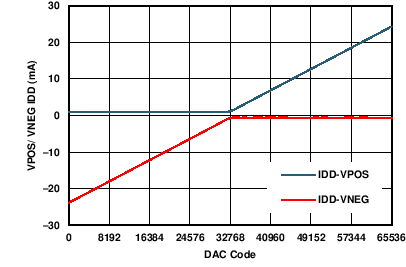±24 mA Range
Figure 21. IOUT Power Supply Current vs Digital Input Code|VPOS_IN_x| = |VNEG_IN_x|, ±24 mA Range, Mid Scale Code
Figure 23. IOUT Power Supply Current vs Power Supplies Voltages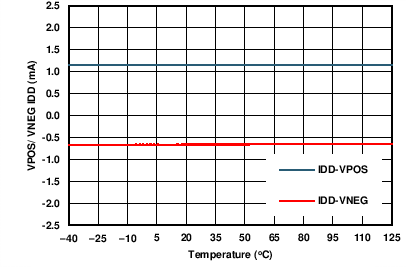±24 mA Range, Mid Scale Code
Figure 22. IOUT Power Supply Current vs Temperature
AVDD/PVDD_x/VPOS_IN_x = +15 V, VNEG_IN_x = –15 V, PBKG = PVSS_x = 0 V, External DVDD = 5 V VOUT disabled, IOUT RL = 250 Ω, TA = 25℃, REFIN = +5 V external, Buck-Boost Converter disabled, unless otherwise stated.0-24 mA Range
Figure 24. IOUT Full-Scale Settling Time, Rising Edge0-24 mA Range
Figure 26. IOUT Full-Scale Settling Time, Falling Edge0-24 mA Range, 7FFFh - 8000h
Figure 28. IOUT Glitch Impulse, Rising Edge, 1LSB Step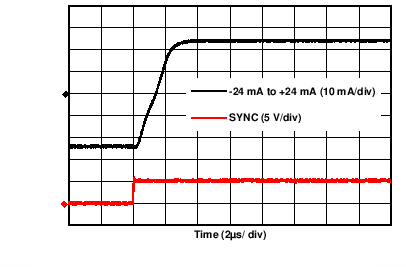AVDD/PVDD_x/VPOS_IN_x = +18 V, VNEG_IN_x = –18 V, IOUT RL = 625 Ω
Figure 25. IOUT Full-Scale Settling Time, Rising EdgeAVDD/PVDD_x/VPOS_IN_x = +18 V, VNEG_IN_x = –18 V, IOUT RL = 625 Ω
Figure 27. IOUT Full-Scale Settling Time, Falling Edge0-24 mA Range, 8000h - 7FFFh
Figure 29. IOUT Glitch Impulse, Falling Edge, 1LSB Step
AVDD/PVDD_x/VPOS_IN_x = +15 V, VNEG_IN_x = –15 V, PBKG = PVSS_x = 0 V, External DVDD = 5 V VOUT disabled, IOUT RL = 250 Ω, TA = 25℃, REFIN = +5 V external, Buck-Boost Converter disabled, unless otherwise stated.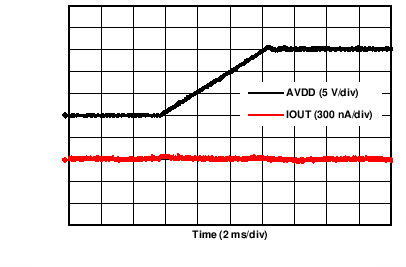Figure 30. IOUT Power-On Glitch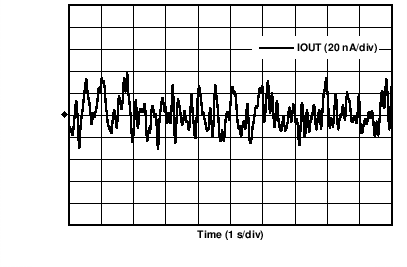0-24mA Range, Mid Scale Code
Figure 32. IOUT Noise, 0.1 Hz to 10 Hz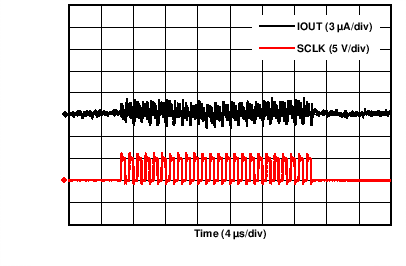0-24 mA Range, Mid Scale Code, SCLK = 1 MHz
Figure 34. Clock Feedthrough IOUT, 1MHz0-24 mA Range
Figure 31. IOUT Enable Glitch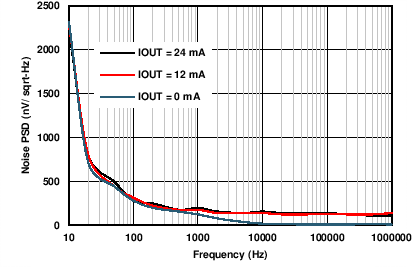0-24 mA Range
Figure 33. IOUT Noise Density vs Frequency
AVDD/PVDD_x = +15 V, PBKG = PVSS_x = 0 V, External DVDD = 5 V, VOUT disabled, IOUT RL = 250 Ω, TA = 25℃, REFIN = +5 V external, Buck-Boost Converter enabled (Full Tracking Mode), unless otherwise stated.Figure 35. IOUT Linearity Error vs Digital Input CodeFigure 37. IOUT Total Unadjusted Error vs Digital Input Code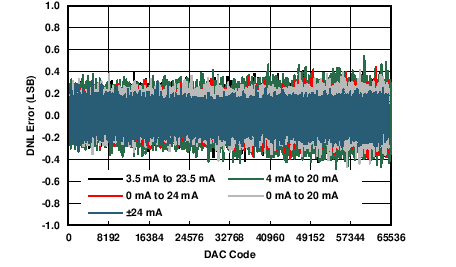Figure 36. IOUT Differential Linearity Error vs Digital Input Code
AVDD/PVDD_x = +15 V, PBKG = PVSS_x = 0 V, External DVDD = 5 V, VOUT disabled, IOUT RL = 250 Ω, TA = 25℃, REFIN = +5 V external, Buck-Boost Converter enabled (Full Tracking Mode), unless otherwise stated.0-24 mA Range
Figure 38. IOUT Full-Scale Settling Time, Rising Edge0-24 mA Range
Figure 40. IOUT Full-Scale Settling Time, Falling Edge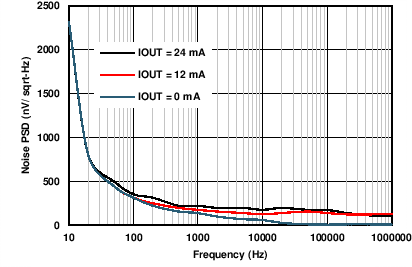0-24 mA Range
Figure 42. IOUT Noise Density vs Frequency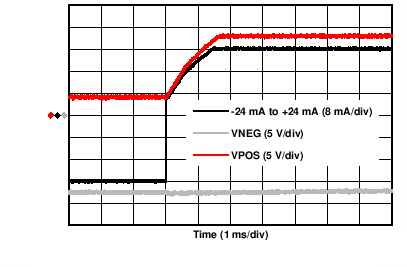IOUT RL = 625 Ω
Figure 39. IOUT Full-Scale Settling Time, Rising Edge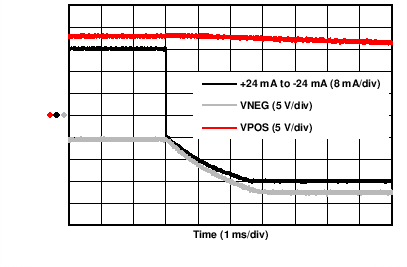IOUT RL = 625 Ω
Figure 41. IOUT Full-Scale Settling Time, Falling Edge0-24 mA Range, Mid Scale Code
Figure 43. IOUT Ripple
AVDD/PVDD_x/VPOS_IN_x = +15 V, VNEG_IN_x = –15 V, VSENSEN_x = PBKG = PVSS_x = 0 V, External DVDD = 5 V. VOUT No load, IOUT disabled; TA = 25℃, REFIN = +5 V external;, Buck-Boost Converter disabled unless otherwise stated.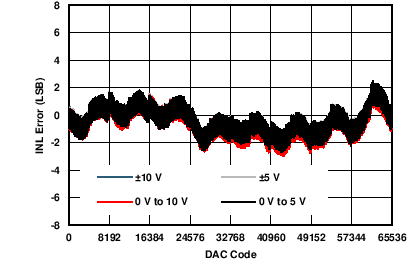Figure 44. VOUT Linearity Error vs Digital Input CodeFigure 46. VOUT Total Unadjusted Error vs Digital Input Code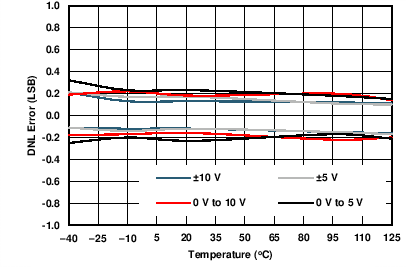Figure 48. VOUT Differential Linearity Error vs Temperature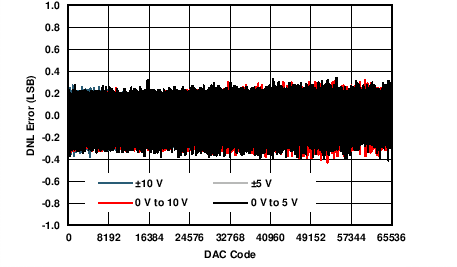Figure 45. VOUT Differential Linearity Error vs Digital Input Code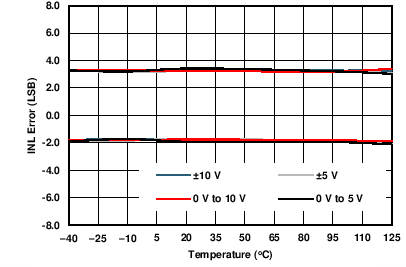Figure 47. VOUT Linearity Error vs Temperature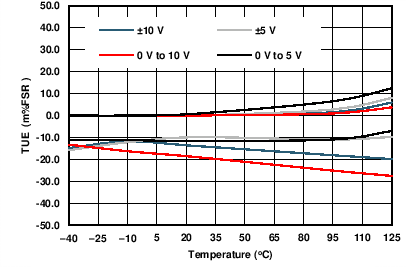Figure 49. VOUT Total Unadjusted Error vs Temperature
AVDD/PVDD_x/VPOS_IN_x = +15 V, VNEG_IN_x = –15 V, VSENSEN_x = PBKG = PVSS_x = 0 V, External DVDD = 5 V. VOUT No load, IOUT disabled; TA = 25℃, REFIN = +5 V external; Buck-Boost Converter disabled unless otherwise stated.Figure 50. VOUT Gain Error vs Temperature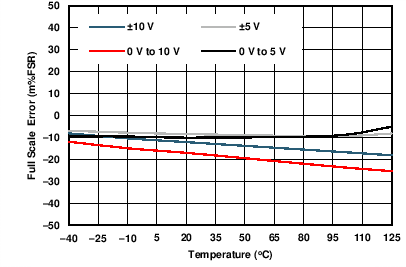Figure 52. VOUT Full Scale Error vs TemperatureFigure 54. VOUT Negative Full Scale Error vs TemperatureFigure 51. VOUT Zero Code Error vs TemperatureFigure 53. VOUT Bipolar Zero Error vs Temperature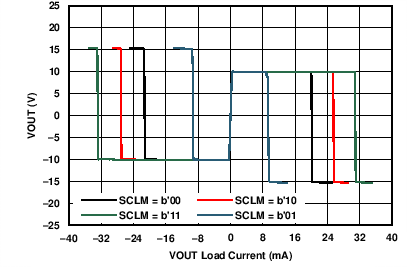±10-V Range, Full Scale Code for VOUT sourcing & Zero Scale Code for VOUT Sinking
Figure 55. VOUT Output Voltage vs Load Current
AVDD/PVDD_x/VPOS_IN_x = +15 V, VNEG_IN_x = –15 V, VSENSEN_x = PBKG = PVSS_x = 0 V, External DVDD = 5 V. VOUT No load, IOUT disabled; TA = 25℃, REFIN = +5 V external; Buck-Boost Converter disabled unless otherwise stated.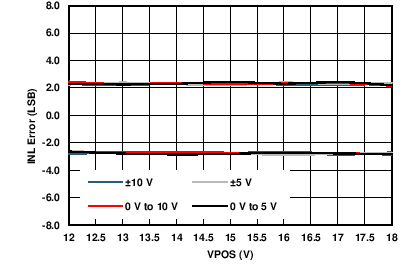|VPOS_IN_x| = VNEG_IN_x
Figure 56. VOUT Linearity Error vs Power Supplies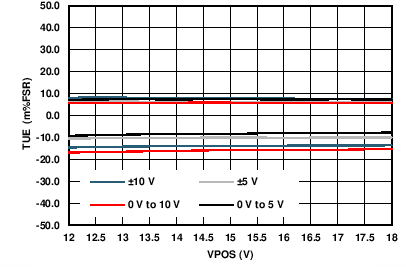|VPOS_IN_x| = VNEG_IN_x
Figure 58. VOUT Total Unadjusted Error vs Power Supplies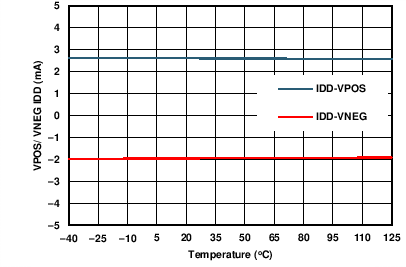|VPOS_IN_x| = VNEG_IN_x, 10-V Range, Mid Scale Code
Figure 60. VOUT Power Supply Current vs Temperature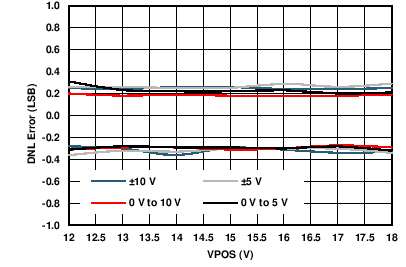|VPOS_IN_x| = VNEG_IN_x
Figure 57. VOUT Differential Linearity Error vs Power Supplies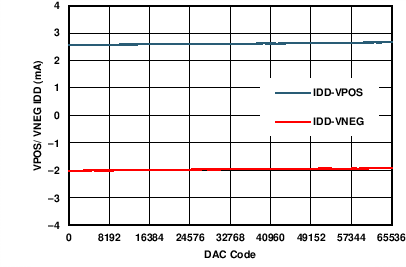|VPOS_IN_x| = VNEG_IN_x, 10-V Range
Figure 59. VOUT Power Supply Current vs Digital Input Code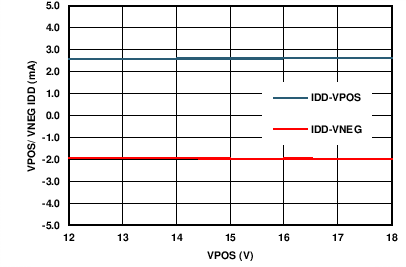|VPOS_IN_x| = VNEG_IN_x, 10-V Range, Mid Scale Code
Figure 61. VOUT Power Supply Current vs Power Supplies Voltages
AVDD/PVDD_x/VPOS_IN_x = +15 V, VNEG_IN_x = –15 V, VSENSEN_x = PBKG = PVSS_x = 0 V, External DVDD = 5 V. VOUT No load, IOUT disabled; TA = 25℃, REFIN = +5 V external;, Buck-Boost Converter disabled unless otherwise stated.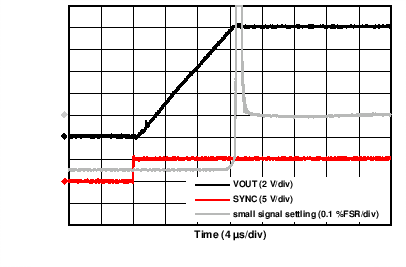Figure 62. VOUT Full-Scale Settling Time, Rising Edge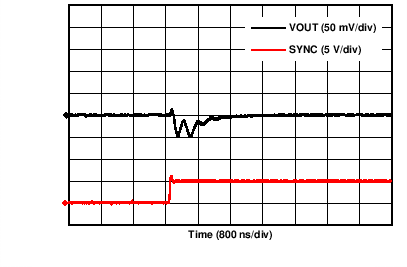10-V Range, 7FFFh - 8000h
Figure 64. VOUT Glitch Impulse, Rising Edge, 1LSB StepFigure 66. VOUT Power-On Glitch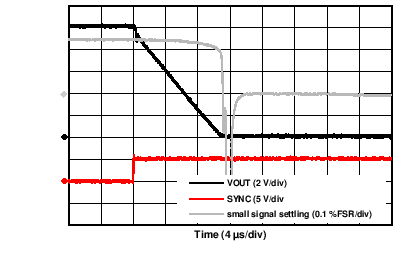Figure 63. VOUT Full-Scale Settling Time, Falling Edge10-V Range, 8000h - 7FFFh
Figure 65. VOUT Glitch Impulse, Falling Edge, 1LSB Step10V Range
Figure 67. VOUT Enable Glitch
AVDD/PVDD_x/VPOS_IN_x = +15 V, VNEG_IN_x = –15 V, VSENSEN_x = PBKG = PVSS_x = 0 V, External DVDD = 5 V. VOUT No load, IOUT disabled; TA = 25℃, REFIN = +5 V external; Buck-Boost Converter disabled unless otherwise stated.10-V Range, Mid Scale Code
Figure 68. VOUT Noise, 0.1 Hz to 10 Hz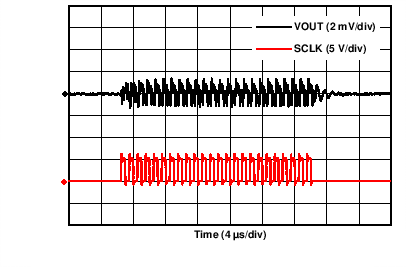10-V Range, Mid Scale Code, SCLK = 1MHz
Figure 70. Clock Feedthrough VOUT, 1MHz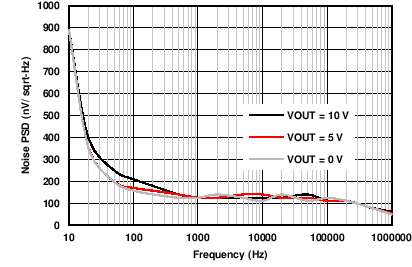10-V Range
Figure 69. VOUT Noise Density vs Frequency
AVDD/PVDD_x = +15 V, VSENSEN_x = PBKG = PVSS_x = 0 V, External DVDD = 5 V. VOUT No load, IOUT disabled; TA = 25℃, REFIN = +5 V external; Buck-Boost Converter enabled (Full Tracking Mode), unless otherwise stated.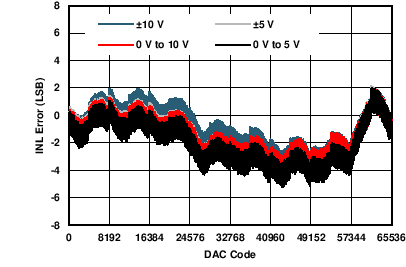Figure 71. VOUT Linearity Error vs Digital Input CodeFigure 73. VOUT Total Unadjusted Error vs Digital Input Code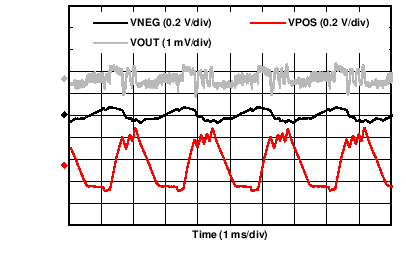10-V Range, Mid Scale Code
Figure 75. VOUT RippleFigure 72. VOUT Differential Linearity Error vs Digital Input Code10-V Range
Figure 74. VOUT Noise Density vs Frequency
AVDD/PVDD_x/VPOS_IN_x = +15 V, VNEG_IN_x = –15 V, PBKG = PVSS_x = 0 V, External DVDD = 5 V. VOUT disabled, IOUT disabled, TA = 25℃, Buck-Boost Converter disabled, unless otherwise stated.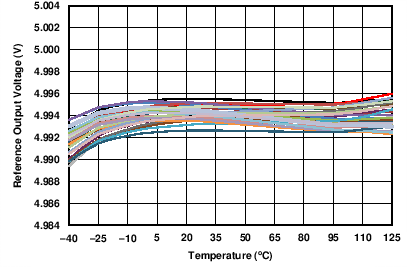30 Units
Figure 76. Internal Reference Voltage vs Temperature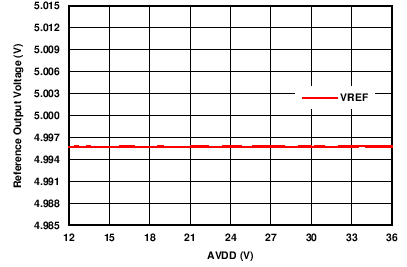Figure 78. Internal Reference Voltage vs Power Supply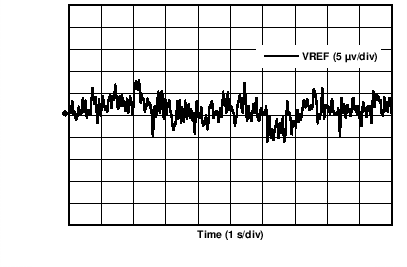Figure 80. Internal Reference Noise, 0.1 Hz to 10 Hz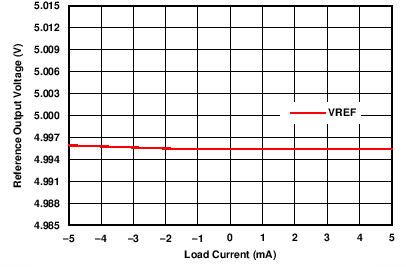Figure 77. Internal Reference Voltage vs Load CurrentFigure 79. Internal Reference Noise Density vs Frequency
AVDD/PVDD_x = +15 V, PBKG = PVSS_x = 0 V, External DVDD = 5 V, VOUT disabled, IOUT RL = 250Ω, TA = 25℃, Buck-Boost Converter enabled (Full Tracking Mode), unless otherwise stated.0-24 mA Range, Full Scale Code on all channels
Figure 81. Internal Reference Noise Density vs Frequency0-24 mA Range, Full Scale Code on all channels
Figure 82. Internal Reference Ripple
AVDD/PVDD_x = +15 V, PBKG = PVSS_x = 0 V, External DVDD = 5 V, TA = 25℃, Buck-Boost Converter enabled (Full Tracking Mode), unless otherwise stated.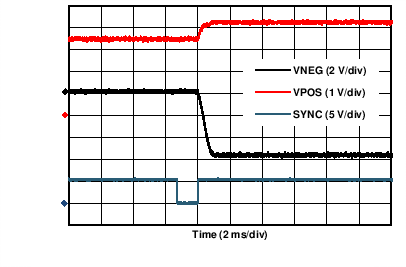Figure 83. Buck-Boost Converter Power-On (IOUT Mode)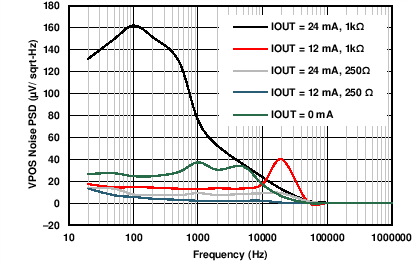0-24 mA Range, RL = 250 Ω
Figure 85. VPOS Noise Density (IOUT Mode) vs Frequency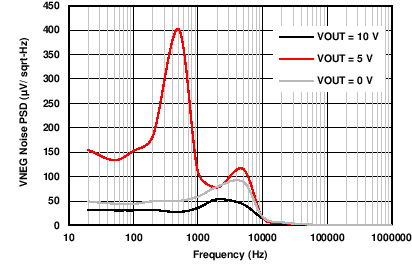Figure 87. VNEG Noise Density (VOUT Mode) vs FrequencyFigure 84. Buck-Boost Converter Power-On (VOUT Mode)Figure 86. VPOS Noise Density (VOUT Mode) vs Frequency
AVDD/PVDD_x = +15 V, VNEG_IN_x = PBKG = PVSS_x = 0 V, External DVDD = 5 V, VOUT disabled, IOUT enabled 0-24 mA Range, TA = 25℃, REFIN = +5 V external, Buck-Boost Converter VPOS_IN_x enabled (Full Tracking Mode), unless otherwise stated.Figure 88. IOUT Efficiency vs Load Current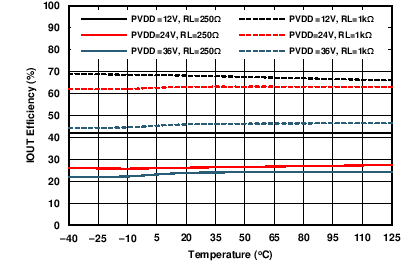Full Scale Code IOUT = 24 mA
Figure 90. IOUT Efficiency vs Temperature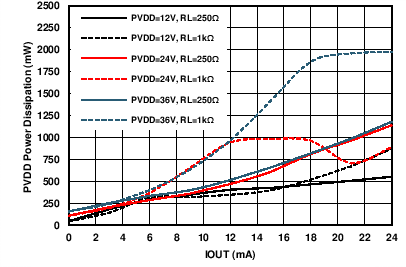Full Scale Code IOUT = 24 mA
Figure 92. PVDD Power Loss (IOUT Mode) vs Load Current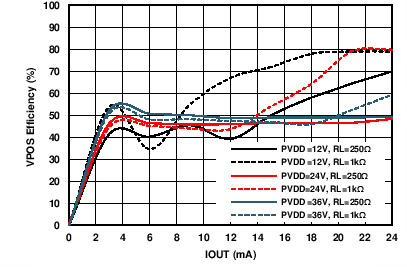Figure 89. VPOS Efficiency (IOUT Mode) vs Load Current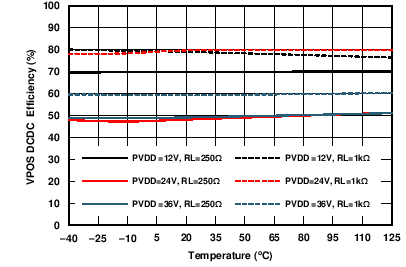Full Scale Code IOUT = 24 mA
Figure 91. VPOS Efficiency (IOUT Mode) vs Temperature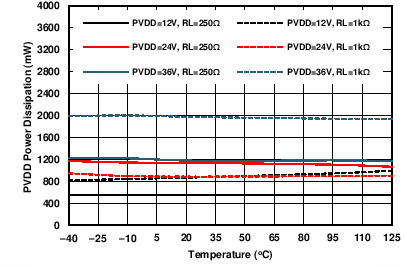Full Scale Code, 24 mA on all channels
Figure 93. PVDD Power Loss (IOUT Mode) vs Temperature
AVDD/PVDD_x = +15 V, PBKG = PVSS_x = 0 V, External DVDD = 5 V, VOUT enabled, 10-V Range, Load 1K//200pF, IOUT disabled, TA = 25℃, REFIN = +5 V external, Buck-Boost Converter enabled (Full Tracking Mode), unless otherwise stated.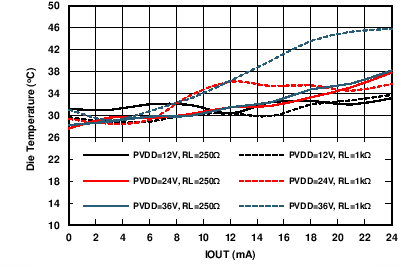VOUT disabled, IOUT = 24 mA (all channels), VNEG_IN_x = 0 V
Figure 94. Intenal Die Temperature (IOUT Mode) vs Load Current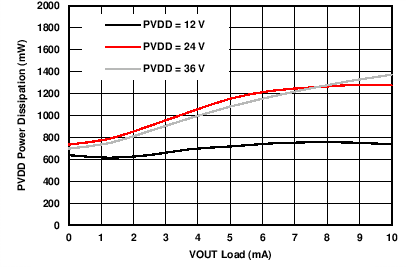Full Scale Code on all channels
Figure 96. PVDD Power Loss (VOUT Mode) vs Load Current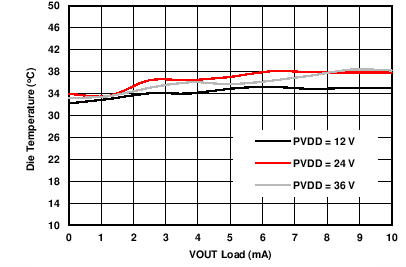All channels enabled
Figure 98. Internal Die Temperature (VOUT Mode) vs Load Current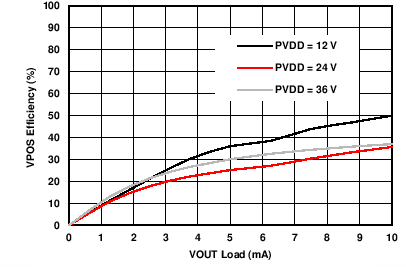Figure 95. VPOS Efficiency (VOUT Mode) vs Load CurrentFull Scale Code on all channels
Figure 97. PVDD Power Loss (VOUT Mode) vs Temperature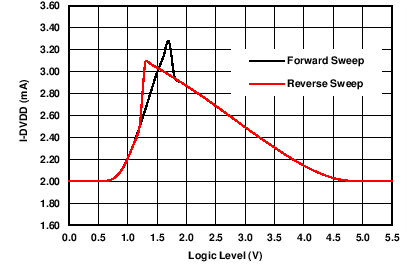Figure 99. Power Supply Current (DVDD) vs Input Logic Level